# CAT 2021 Slot 1

## CAT 2021 Exam Question Papers Slot 1 | CAT Quants​

### CAT Quantitative Aptitude | CAT 2021 Question Papers​

CAT Question Papers: Solving CAT Question Papers from prior years will give CAT 2022 applicants invaluable insight into the examination format, question pattern, and level of difficulty. The CAT 2021 exams were held in three slots.

It is important for CAT preparation to examine old CAT Exam, Question Paper. In order to overcome the difficulties, they encounter when preparing for the CAT, aspirants must use a variety of strategies.
• By solving CAT Exam previous year question papers, aspirants learn about their own strengths and weaknesses. This allows them to plan their preparation accordingly.
• The secret to passing any exam is practice. Hence, by studying CAT exam papers over the last 5 to 10 years, you can attempt 500+ questions of all types and levels of difficulty.
• They can identify important topics by asking questions that have been asked repeatedly over time.
• CAT aspirants can benefit from numerous study materials and engaging video lessons available at Pathfinder For Me, including Free Download CAT Exam E-Books. Aspirants for the CAT test will get closer to passing the CAT 2022 exam with the help of these CAT question papers with solution PDF.

The best resource for CAT preparation is CAT question papers. Solving previous year’s CAT question papers helps candidates understand the CAT Exam Pattern, types of questions, question weightage, important topics, etc.

All CAT 2021 Quantitative aptitude questions have detailed answer keys and solutions provided by our experts. Watch the videos below to learn the fastest ways to answer the questions:

1).

Amal purchases some pens at ₹ 8 each. To sell these, he hires an employee at a fixed wage. He sells 100 of these pens at ₹ 12 each. If the remaining pens are sold at ₹ 11 each, then he makes a net profit of ₹ 300, while he makes a net loss of ₹ 300 if the remaining pens are sold at ₹ 9 each. The wage of the employee, in INR, is

2).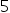-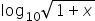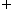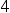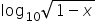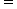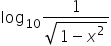, then 100x equals

3).

f(x) =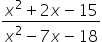is negative if and only if

1. -5 < x < -2 or 3 < x < 9

2. -2 < x < 3 or x > 9

3. x < -5 or 3 < x < 9

4. x < -5 or -2 < x < 3

4).

The number of integers n that satisfy the inequality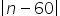<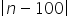<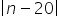is

1. 21

2. 18

3. 20

4. 19

5).

Identical chocolate pieces are sold in boxes of two sizes, small and large. The large box is sold for twice the price of the small box. If the selling price per gram of chocolate in the large box is 12% less than that in the small box, then the percentage by which the weight of chocolate in the large box exceeds that in the small box is nearest to

1. 127

2. 135

3. 124

4. 144

6).

A basket of 2 apples, 4 oranges and 6 mangoes costs the same as a basket of 1 apple, 4 oranges and 8 mangoes, or a basket of 8 oranges and 7 mangoes. Then the number of mangoes in a basket of mangoes that has the same cost as the other baskets is

1. 12

2. 13

3. 11

4. 10

7).

If the area of a regular hexagon is equal to the area of an equilateral triangle of side 12 cm, then the length in cm of each side of the hexagon is equal to

1. 4√6

2. 6√6

3. 2√6

4. √6

8).

Onion is sold for 5 consecutive months at the rate of Rs. 10, 20, 25, 25, and 50 per kg, respectively. A family spends a fixed amount of money on onion for each of the first three months, and then spends half that amount on onion for each of the next two months. The averageexpense for onion, in rupees per kg, for the family over these 5 months is closest to

1. 18

2. 26

3. 16

4. 20

9).

If r is a constant such that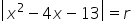has exactly three distinct real roots, then the value of r is

1. 15

2. 18

3. 17

4. 21

10).

Anil invests some money at a fixed rate of interest, compounded annually. If the interests accrued during the second and third year are ₹ 806.25 and ₹ 866.72, respectively, the interest accrued, in INR, during the fourth year is nearest to

1. Rs 931.72

2. Rs 926.84

3. Rs 929.48

4. Rs 934.65